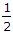# Civil Engineering - Applied Mechanics - Discussion

### Discussion :: Applied Mechanics - Section 1 (Q.No.23)

23.

The masses of two balls are in the ratio of 2 : 1 and their respective velocities are in the ratio of 1 : 2 but in opposite direction before impact. If the coefficient of restitution is, the velocities of separation of the balls will be equal to

 [A]. original velocity in the same direction [B]. half the original velocity in the same direction [C]. half the original velocity in the opposite direction [D]. original velocity in the opposite direction

Explanation:

No answer description available for this question.

 Hari Har said: (Jul 21, 2016) Someone please explain this to me.

 Snehal Wankhede said: (Aug 30, 2016) I think it's option C. Using, 1) m1v1 + m2v2 = m1v1' + m2v2'. 2) e = -(v2' - v1')/(v2 - v1). Answer v2' = -v2/2, v1' = -v1/w2.

 Kush Kakkar said: (Jan 17, 2017) Coefficient of restitution = Relative velocity before collision / relative velocity after collision 1:2 = 1:2/ relative velocity after collision. The relative velocity after collision = (1:1).

 Asha said: (May 9, 2017) I think Option C is correct.

 Lasith said: (Jul 27, 2018) The mass of 1st ball (m1)=2M, mass of 2nd ball (m2)= M initial velocity of 1st ball (u1)= U, initial velocity of 2nd ball (u2)= -2U { -ve sign because of opp direction. The coefficient of restitution=1/2, let, v1 = final velocity of 1st ball, v2= final velocity of the 2nd ball from the law of conservation of momentum, m1u1+m2u2=m1v1+m2v2. 2MU+M(-2U)=2Mv1+Mv2. 0=2Mv1+Mv2. v2=-2v1. From the law of collision of elastic bodies that; (v2-v1)=e(u1-u2). On solving v1=-1/2 U. So negative sign indicates that the direction of v1 is opposite to that of U thus 1st ball will move back with 1/2 of its original velocity.

 Keshav said: (Jun 17, 2019) C should be the answer, since v2-v1= -u/2 ie half the original velocity of the first ball in opposite direction.